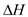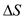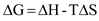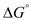# Quiz 7: Metabolism and Energetics

Biology

The enthalpy changeof reaction is 30 kJ/mol or 30000 J/mol The entropy changeof the system is 100 J/mol The temperature is 300 K The Gibbs free energy equation is as follows:Substitute the given values:Hence, the reaction is at equilibrium because the Gibbs free energy change is zero. If the temperature of the reaction would have been higher, then the free energy change would be in negative value; hence, the reaction would have become exergonic (heat energy releasing).

The free energy change of a system that takes place in a reaction can be measured under different set of conditions. If the given conditions are at standard-state, then the result is the standard-state free energy () of reaction. When the free energy is zero that is= 0 then the reaction is at equilibrium. At this stage, the equilibrium constant (K eq ) equals the mass action ratio (Q). The determination of the reaction under cellular conditions is not possible by either equilibrium constant or by standard conditions.

Internal energy is the energy that is involved in the molecule. The enthalpy is the energy of bond formation or bond breakage which is also an internal energy of the molecule. Thus, internal energy and enthalpy are similar. The enthalpy is still retained in the free energy change because it is involved in the changes that occur during chemical changes of a reaction. In the human body, the chemical reaction keep on occurring and ultimately cause enthalpy change thus, it is still included in the free energy change.